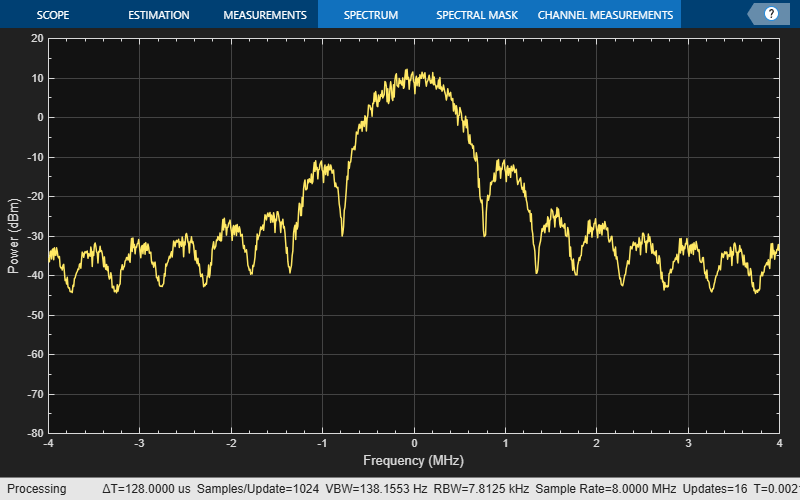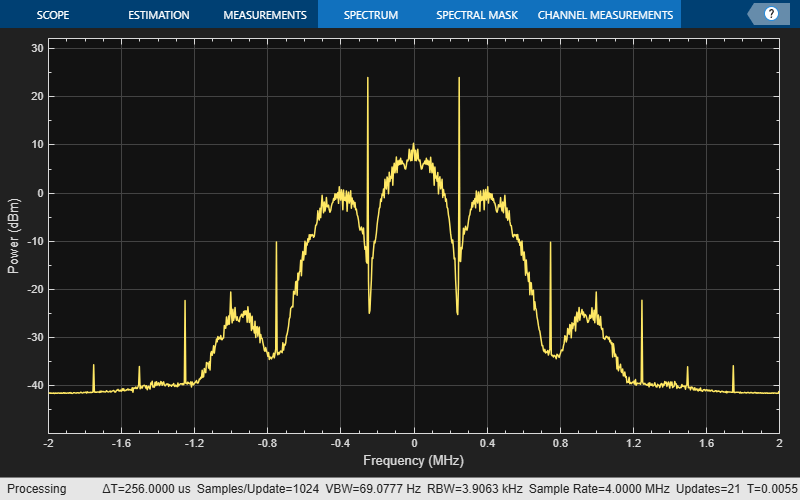# bleWaveformGenerator

Generate Bluetooth LE PHY waveform

## Syntax

``waveform = bleWaveformGenerator(message)``
``waveform = bleWaveformGenerator(message,Name,Value)``

## Description

example

````waveform = bleWaveformGenerator(message)` generates `waveform`, a time-domain Bluetooth® low energy (LE) physical layer (PHY) waveform by using the input information bits, `message`.```

example

````waveform = bleWaveformGenerator(message,Name,Value)` also specifies options using one or more name-value pair arguments. For example, `'Mode','LE2M'` specifies the generating mode value of the desired Bluetooth LE waveform.```

## Examples

collapse all

Create an input message column vector of length 2056 containing random binary values. Set the symbol rate to default value.

```message = randi([0 1],2056,1); symbolRate = 1e6;```

Generate the Bluetooth LE waveform.

`waveform = bleWaveformGenerator(message);`

Create a spectrum analyzer System object to display the frequency spectrum of the generated Bluetooth LE waveform. Set the sample rate of the frequency spectrum.

```scope = spectrumAnalyzer; scope.SampleRate = 8*symbolRate;```

Plot the Bluetooth LE waveform.

`scope(waveform);`Create an input message column vector of length 640 containing random binary values.

`message = randi([0 1],640,1);`

Specify the values of generating mode, channel index, samples per symbol and access address. Set symbol rate to default value.

```phyMode = "LE125K"; chanIdx = 2; sps = 4; accAdd = [1 1 1 1 0 1 0 0 1 1 0 1 0 0 1 0 0 1 1 0 1 1 1 0 1 ... 0 1 0 1 1 0 0].'; symbolRate = 1e6;```

Create a spectrum analyzer System object to display the frequency spectrum of the generated Bluetooth LE waveform. Set the sample rate of the frequency spectrum.

```scope = spectrumAnalyzer; scope.SampleRate = sps*symbolRate;```

Generate and visualize the Bluetooth LE waveform.

```waveform = bleWaveformGenerator(message,'Mode',phyMode, ... 'SamplesPerSymbol',sps,'ChannelIndex',chanIdx,'AccessAddress',accAdd); scope(waveform);```Specify a connection data channel protocol data unit (PDU) for angle of departure (AoD) constant tone extension (CTE).

```pduHex = '1B820127'; % Valid PDU in hexadecimal pdu = de2bi(hex2dec(pduHex), 32)';```

Generate and append cyclic redundancy check (CRC) to the PDU.

```crcGen = comm.CRCGenerator('z^24+z^10+z^9+z^6+z^4+z^3+z+1', ... 'InitialConditions',de2bi(hex2dec('555551'),'left-msb',24), ... 'DirectMethod',true); pduCRC = crcGen(pdu);```

Generate the Bluetooth LE waveform.

```waveform = bleWaveformGenerator(pduCRC,'ChannelIndex',36,... 'DFPacketType','ConnectionCTE');```

## Input Arguments

collapse all

Input message bits, specified as a binary-valued column vector of numerical or logical values. This input contains the protocol data unit (PDU) and cyclic redundancy check (CRC) data. The maximum length of this value is 2088 bits.

Data Types: `double` | `logical`

### Name-Value Arguments

Specify optional pairs of arguments as `Name1=Value1,...,NameN=ValueN`, where `Name` is the argument name and `Value` is the corresponding value. Name-value arguments must appear after other arguments, but the order of the pairs does not matter.

Before R2021a, use commas to separate each name and value, and enclose `Name` in quotes.

Example: `bleWaveformGenerator(message,'Mode','LE2M','ChannelIndex',36)`

Note

For information about connection CTE and connectionless CTE direction finding packet generation, see Bluetooth Packet Structure.

PHY generating mode, specified as the comma-separated pair consisting of `'Mode'` and `'LE1M'`, `'LE2M'`, `'LE500K'`, or `'LE125K'`.

Data Types: `char` | `string`

Channel index, specified as the comma-separated pair consisting of `'ChannelIndex'`and an integer in the range [0, 39]. For data channels, this value must be in the range [0, 36]. The data-whitening block uses this value to randomize the bits.

Data Types: `double`

Samples per symbol, specified as the comma-separated pair consisting of `'SamplesPerSymbol'` and a positive integer. The function uses this value for the Gaussian frequency-shift keying (GFSK) modulation.

Data Types: `double`

Access address, specified as the comma-separated pair consisting of `'AccessAddress'` and a 32-bit column vector of numerical or logical values.

Data Types: `double` | `logical`

Type of direction finding packet, specified as the comma-separated pair consisting of `'DFPacketType'` and `'ConnectionlessCTE'`, `'ConnectionCTE'`, or `'Disabled'`.

Data Types: `char` | `string`

Data whiten status, specified as `'On'` or `'Off'`. To perform whitening on message, set this value to `'On'`.

Data Types: `char` | `string`

## Output Arguments

collapse all

Output time-domain waveform, returned as a complex-valued column vector of size Ns-by-1, where Ns represents the number of time-domain samples. The function returns this output in the form of complex in-phase quadrature (IQ) samples.

Data Types: `double`

 Bluetooth Technology Website. “Bluetooth Technology Website | The Official Website of Bluetooth Technology.” Accessed November 22, 2021. https://www.bluetooth.com/.

 Bluetooth Special Interest Group (SIG). "Bluetooth Core Specification." Version 5.3. https://www.bluetooth.com/.